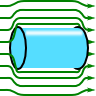## Superconductors and Their Magnetostatic FieldsThe Proca-formulation of electrodynamics allows to account for a hypothetical massive photon. This formulation lead to astonishing experiments but is formally equivalent to the London theory of superconductivity. Learn in this problem how a magnetic field is expelled from a superconductor by "massive photons".

## Problem StatementA superconducting half-plane is terminated at $$z=0$$. Outside of the superconductor, there is a magnetostatic field $$\mathbf{B}\left(\mathbf{r}\right)=B_{0}\mathbf{e}_{y}$$ parallel to the surface. Inside the superconductor, the Proca equation $\left(\Delta-\mu_{\mathrm{L}}^{2}\right)\mathbf{A}\left(\mathbf{r}\right) = 0$ shall be applicable.
Calculate the magnetic induction with respect to the London penetration depth $$\lambda_{\mathrm{L}}=1/\mu_{\mathrm{L}}$$. Now consider the case if the superconductor is a slab with a finite thickness $$d$$, centered at $$z=0$$. What does the induction look like now? Calculate an effective current $$\mathbf{j}\left(\mathbf{r}\right)$$ inside the superconductor consistent with the calculated magnetic induction. Discuss qualitatively how the magnetic field and current should look like if one replaces the superconducting slab with a superconducting wire of some radius.

## Background: Photon Mass and Superconductivity

If photons had a nonvanishing mass, the electromagnetic fields would show different characteristics than those we are used to: a wavelength dependence of the speed of light, modifications of Coulomb's and Ampères law and thus different fields for charges and dipoles and so on. Without a doubt, the question for a photon mass is very fundamental. Here, however, we can use it to explain superconductivity following the Londons. For much more information please have a look at The "Mass" of the Photon - Magnetic Fields in Superconductors.